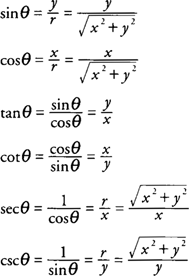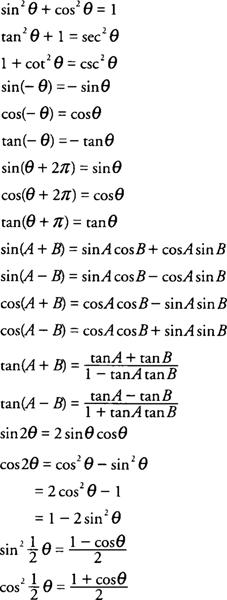## Trigonometric Functions

In trigonometry, angle measure is expressed in one of two units: degrees or radians. The relationship between these measures may be expressed as follows: 180° = π radians.

To change degrees to radians, the equivalent relationship 1° = π/180 radians is used, and the given number of degrees is multiplied by π/180 to convert to radian measure. Similarly, the equation 1 radian = 180°/π is used to change radians to degrees by multiplying the given radian measure by 180/π to obtain the degree measure.

The six basic trigonometric functions may be defined using a circle with equation x 2 + y 2 = r 2 and the angle θ in standard position with its vertex at the center of the circle and its initial side along the positive portion of the x‐axis (see Figure ).Figure 1 Defining the trigonometric functions.

The trigonometric functions sine, cosine, tangent, cotangent, secant, and cosecant are defined as follows:It is essential that you be familiar with the values of these functions at multiples of 30°, 45°, 60°, 90°, and 180° (or in radians, π/6, π/4, π/3, π/2, and π (See Table .) You should also be familiar with the graphs of the six trigonometric functions. Some of the more common trigonometric identities that are used in the study of calculus are as follows:The relationship between the angles and sides of a triangle may be expressed using the Law of Sines or the Law of Cosines (see Figure 2).Figure 2 Relations between sides and angles of a triangle.